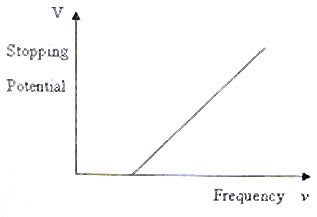## Pages

`“Life is like riding a bicycle.  To keep your balance you must keep moving.”–Albert Einstein`

## Saturday, March 1, 2008

### AP Physics B – Additional Questions (MCQ) on Atomic Physics and Quantum Effects

In continuation of the post dated 25 February 2008, I give below a few more questions on atomic physics and quantum effects

(1) An electron and a proton have the same wave length. Which one of the following statements is correct about them?

(a) The momentum of the proton is less than that of the electron

(b) The momentum of the proton is greater than that of the electron

(c) They have the same speed

(d) The kinetic energy of the proton is greater than that of the electron

(e) The kinetic energy of the proton is less than that of the electron

We have de Broglie wave length λ = h/p.

Since ‘h’ is Planck’s constant, the momentum ‘p’ must be the same. The kinetic energy (K) is given by

K = p2/2m where ‘m’ is the mass.

Since the mass of the proton is greater than that of the electron, the kinetic energy of the proton must be less than that of the electron.

(2) A stationary nucleus of mass M emits an electron of mass ‘m’ with a velocity ‘v’. If the recoil velocity of the nucleus is V, the ratio of the de Broglie wave lengths of the nucleus and the electron is

(a) M/m

(b) (M­ m)/m

(c) V/v

(d) v/V

(e) 1

This is a very simple question. By the law of conservation of momentum, the momentum (p) of the electron is equal in magnitude (and opposite in direction) to the recoil momentum of the nucleus.

Since the wave length, λ = h/p, they have the same wave length and the ratio of wave lengths is 1 [Option (e)].

(3) In an experiment on photo electric effect, a photoelectric target is irradiated with laser beams of various frequencies and in each case the stopping potential is measured. On using the stopping potentials as the Y- coordinates and the frequencies as the X- coordinates, a straight line graph is obtained. If Ф is the work function, ‘e’ is the electronic charge and ‘h’ is Planck’s constant, the slope of this straight line is equal to

(a) h/e

(b) h

(c) Ф

(d) Ф/e

(e) Ф/h

The graph will be as shown in the adjoining figure. Einstein’s photoelectric equation is

= Ф + Kmax where ν is the frequency of the incident light and Kmax is the maximum kinetic energy of the photo electrons, which we can write in terms of the stopping potential Vs as

Kmax = eVs.

Therefore, we have

= Ф + eVs so that Vs = (h/e) ν Ф/e

This equation is of the form y = mx +c which is the equation of a straight line of slope ‘m’. Therefore, on plotting the stopping potential Vs against the frequency ν, a straight line of slope h/e is obtained.

(4) If an antimatter world exists, hydrogen atoms (to be precise, anti hydrogen atoms) there would be made of positrons revolving round anti protons. If the kinetic energy of the positron in the first orbit (n = 1) of such an anti hydrogen atom is K, what will be the total energy of the positron in the third orbit?

(a) K

(b) – K

(c) – K/3

(d) K/9

(e) – K/9

The motion of the positron will be under the inverse square law force of attraction between the anti proton and the positron and will be similar to the motion of the electron in an ordinary hydrogen atom. The expressions for energies will be the same as in the case of an ordinary hydrogen atom. The kinetic energy in any orbit will be positive where as the total energy will be negative but they will be of the same amount.

Since the energy is inversely proportional to the square of the quantum number ‘n’, the total energy in the third orbit will be –K/32 = –K/9.

[Note that all these results are true for an ordinary hydrogen atom].

(5) The Hα line is the first member of the Balmer series of the hydrogen spectrum and it occurs due to the transition of the electron from the 3rd orbit to the 2nd orbit. If its wave length is λ, the wave length of the last member of the Balmer series will be

(a) (4/9) λ

(b) (5/9) λ

(c) (7/9) λ

(d) (1/2) λ

(e) (1/3) λ

On applying Rydberg’s relation to the Hα line, we have

1/λ = R[(1/22) – (1/32)] = (5/36)R where R is Rydberg’s constant.

The last member of the Balmer series is due to the transition from the last orbit (n = ∞) to the 2nd orbit. If its wave length is λl, we have

1/λl = R[(1/22) – (1/2)] = (1/4)R

Dividing the first equation by the second, we have

λl/λ = 20/36

Therefore, λl = (5/9) λ.

You can expect some free response questions in this section shortly.

Meanwhile see some multiple choice questions (with solution) at physicsplus: Questions on Bohr Atom Model.